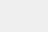# 使用 keras 和 tfjs 构建血细胞分类模型AI 真的是一个重大的游戏改变者。 AI的应用是巨大的，并且它在医疗领域的应用范围也是广阔的。 先进的AI工具可以帮助医生和实验室技术人员更准确地诊断疾病，例如尼日利亚的一名医生可以使用这种工具从血液样本中识别出一种他根本不知道的疾病，这有助于他更好地诊断这种疾病并且更快的治疗，这是AI民主化的一个优势，因为AI模型和工具可以在全世界范围内使用，使得尼日利亚的医生可以使用麻省理工学院或世界上任何其他伟大的大学的研究学者正在使用的工具和技术。

## 机器学习的主要问题

• Kaggle (Home of datasets) : 没有可用的数据集是主要问题之一，但是Kaggle是人们创建数据集并托管以使其他人使用的最佳场所，人们使用这些工具构建了令人有趣的东西。

## 血细胞数据集

Dataset Structure : 该数据集包含了 12,500 张增强的血细胞图片，该数据集包含下列4种不同的类型：### Kaggle 身份验证：

Kaggle 命令行界面允许您下载数据集并将代码提交给竞赛。 注册kaggle后，您可以下载包含所有凭据的kaggle.json文件，kaggle 命令行界面使用这些凭据进行授权。

• 使用命令 `!mkdir .kaggle` 创建一个新单元和一个名为.kaggle的隐藏文件夹。
• 使用pip安装Kaggle CLI ：在新单元中- `!pip install kaggle`
• 下载数据集：`!kaggle datasets download -d paulthimothymooney/blood-cells`
• 确保当前的文件夹包含下载的数据集 `!ls dataset2-master/images`
• 可以看到三个文件夹：TEST, TEST_SIMPLE and TRAIN
• TRAIN 文件夹包含了训练图片，将会使用这个文件夹来进行训练。

### 预处理

```from keras.models import Sequential
from keras.layers import Dense, Conv2D, Dropout, MaxPool2D, Flatten
from keras.preprocessing import image
```

`keras.preprocessing` 提供处理各种类型数据集所需的方法和对象。 从图像模块我们创建一个具有所有必需配置的ImageDataGenerator。

```generator = image.ImageDataGenerator(
rescale = 1./255,
featurewise_center=False,  # set input mean to 0 over the dataset
samplewise_center=False,  # set each sample mean to 0
featurewise_std_normalization=False,  # divide inputs by std of the dataset
samplewise_std_normalization=False,  # divide each input by its std
zca_whitening=False,  # apply ZCA whitening
rotation_range=10,  # randomly rotate images in the range (degrees, 0 to 180)
width_shift_range=0.1,  # randomly shift images horizontally (fraction of total width)
height_shift_range=0.1,  # randomly shift images vertically (fraction of total height)
horizontal_flip=True,  # randomly flip images
vertical_flip=False)
```

```dataset = generator.flow_from_directory(
shuffle = True,
batch_size = 32,
target_size = (80, 80),
directory = 'dataset2-master/images/TRAIN'
)
```

### CNN 简介

CNN（卷积神经网络）是一种神经网络，它包含一组卷积层和一个连接到它的前馈网络。 卷积操作不是一个新的操作，它多年来一直用于图像处理。 卷积操作的主要作用是从图像中提取边缘，换句话说，它们可用于提取图像的重要特征，如果滤波器值已知，则对于任何图像任何人都无法得出最佳滤波器值，因为我们使用卷积和神经网络，梯度下降将自动优化滤波器值以提取图像的重要特征。 Andrew Ng的课程deeplearning.ai可以帮助您更好地理解这些网络的工作。 它超出了本文的范围。

### 网络```def model():
model = Sequential()
model.add(Conv2D(80, (3,3), strides = (1, 1), activation = 'relu'))
model.add(Conv2D(64, (3,3), strides = (1, 1), activation = 'relu', input_shape = (80, 80, 3)))
model.add(Conv2D(64, (3,3), strides = (1,1), activation = 'relu'))

return model
```

```nn = model()
nn.fit_generator(dataset, steps_per_epoch = None, epochs = 30, verbose = 1)
nn.save('Model.h5')
```

## 部署模型

### 先决条件：```npm install -g create-react-app
create-react-app app_name
cd app_name
npm install --save @tensorflow/tfjs
//on server side:
mkdir server
cd server
npm init
//fill up the details, make sure package.json is created
npm install express --save
```
• 模型服务器：这是一个express.js REST终端，客户端可以通过发送REST GET请求来请求模型文件。 （在服务器端）
```let express = require('express')
let cors = require('cors')
let path = require('path')

var app = express()

let static_path = path.join(__dirname, 'ModelData/model_data')

app.use(cors())
app.use(express.static(static_path))
```
• 模型仓库：我们需要创建一个与tfjs兼容的模型，tensorflow提供了一个名为tensorflowjs的工具，它是一个包含实用程序的python工具包，我们可以使用以下命令来安装它：`pip install tensorflowjs`

```\$tensorflowjs_converter --input_format keras \
Model.h5 \
ModelData/model_data
// Model.h5 is the downloaded model after training, last argument is the target folder, where we need to store the model files.
```

### 最后一部分：在客户端开发接口引擎

• 创建一个模型容器类：首先为我们的模型创建了一个包装类。 此类的实例表示可以进行接口的模型。 这个模型类的代码是可以理解的：
• 接口函数：定义了一个可以获取模型对象和输入图像源的函数，输入源可以是HTML img，也可以是URL或图像字节流。

```var tensorflow = require('@tensorflow/tfjs')

class ModelContainer {
constructor(nn){
this.nn = nn
}
setWeights(weights){
//use json weights file
}
console.log(url)
console.log(this.nn)
}
removeNetwork(){
this.nn = null
}

swapToNewObject(){
let new_nn = this.nn
this.nn = null
return new_nn
}

obtainMemorySafe(){
return this.swapToNewObject()
}

obtain(){
return this.nn
}
}

function runInference(modelContainer, imageData){
//perform a sawp, i.e obtain network object from container
imageData.style.width = "80px";
imageData.style.height = "80px";
let nn = modelContainer.obtainMemorySafe()
let pixels = tensorflow.fromPixels(imageData)
pixels = pixels.reshape([1, 80, 80, 3])
pixels.dtype = 'float32'
pixels = tensorflow.div(pixels, 255)
let predictions = nn.predict(pixels)
modelContainer.nn = nn
nn  = null
return predictions.dataSync()
}

function afterPrediction(predictions){
//as of now
console.log(predictions)
}

export {ModelContainer, runInference, afterPrediction}
```
• 初始化模型对象：我们现在可以创建模型对象以便用在接口中。
```let modelCache = new ModelContainer(null);
```
• 运行接口：一旦我们有了一个模型对象，我们就可以随时进行接口。根据我设计的用户界面，只要用户点击预测按钮，就会执行接口。 运行预测的React组件部分如下所示：
```<div style = {{margin : '40px 40px 40px 40px', textAlign : 'center'}}>
<button className = "button is-rounded is-danger" onClick = {
() => {
let result = runInference(modelCache, document.getElementById('image_container'))
this.setState({
scores : this.computeScore(result),
show_result : true
})
}
}>Predict</button>
</div>

//and computeScore function :
computeScore(predictions) {
let sum = 0;
for(var i = 0; i < predictions.length; i++) sum+=predictions[i]

//cumpute proportions
let proportions = []
for(var i = 0; i < predictions.length; i++){
let prop = (predictions[i] * 100)/sum
proportions.push(prop)
}

return {
EOSINOPHIL : proportions,
LYMPHOCYTE : proportions,
MONOCYTE : proportions,
NEUTROPHIL : proportions
}
}
}
```

## 总结

REPO URL : react-client

Cloud Notebook (训练模型)： training.ipynb